# YEAR function convert date to year in Excel

This tutorial explains the syntax and uses of the Excel YEAR function and provides formula examples to extract year from date, convert date to month and year, calculate age from the date of birth and determine leap years.

In a few recent posts, we have explored different ways to calculate dates and times in Excel and learned a variety of useful functions such as WEEKDAY, WEEKNUM, MONTH, and DAY. Today, we are going to focus on a bigger time unit and talk about calculating years in your Excel worksheets.

In this tutorial, you will learn:

## YEAR function in Excel

The YEAR function in Excel returns a four-digit year corresponding to a given date as an integer from 1900 to 9999.

The syntax of the Excel YEAR function is as simple as it could possibly be:

YEAR(serial_number)

Where serial_number is any valid date of the year you want to find.

## Excel YEAR formula

To make a YEAR formula in Excel, you can supply the source date in several ways.

### Using the DATE function

The most reliable way to supply a date in Excel is using the DATE function.

For example, the following formula returns the year for 28 April, 2015:

`=YEAR(DATE(2015, 4, 28))`

### As a serial number representing the date

In the internal Excel system, dates are stored as serial numbers beginning with 1 January 1900, which is stored as number 1. For more information on how dates are stored in Excel, please see Excel date format.

The 28 day of April, 2015 is stored as 42122, so you can enter this number directly in the formula:

`=YEAR(42122)`

Though acceptable, this method is not recommended because date numbering may vary across different systems.

### As a cell reference

Assuming you have a valid date in some cell, you can simply refer to that cell. For example:

`=YEAR(A1)`

### As a result of some other formula

For example, you can use the TODAY() function to extract the year from the current date:

`=YEAR(TODAY())`

### As text

In a simple case, the YEAR formula can even understand dates entered as text, like this:

`=YEAR("28-Apr-2015")`

When using this method, please double check that you enter the date in the format that Excel understands. Also, please remember that Microsoft does not guarantee correct results when a date is supplied as a text value.

The following screenshot demonstrates all of the above YEAR formulas in action, all returning 2015 as you might expect :)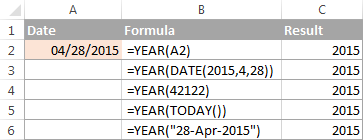## How to convert date to year in Excel

When you work with date information in Excel, your worksheets usually display full dates, including month, day and year. However, for major milestones and important events such as product launches or asset acquisitions, you may want to view only the year without re-entering or modifying the original data. Below, you will find 3 quick ways to do this.

### Example 1. Extract a year from date using the YEAR function

In fact, you already know how to use the YEAR function in Excel to convert a date to a year. The screenshot above demonstrates a bunch of formulas, and you can see a few more examples in the screenshot below. Notice that the YEAR function perfectly understands dates in all possible formats: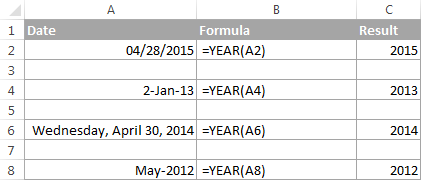### Example 2. Convert date to month and year in Excel

To convert a given date to year and month, you can use the TEXT function to extract each unit individually, and then concatenate those functions within one formula.

In the TEXT function, you can use different codes for months and years, such as:

• "mmm" - abbreviated months names, as Jan - Dec.
• "mmmm" - full month names, as January - December.
• "yy" - 2-digit years
• "yyyy" - 4-digit years

To make the output better readable, you can separate the codes with a comma, hyphen or any other character, like in the following Date to Month and Year formulas:

`=TEXT(B2, "mmmm") & ", " & TEXT(B2, "yyyy")`

Or

`=TEXT(B2, "mmm") & "-" & TEXT(B2, "yy")`

Where B2 is a cell containing a date.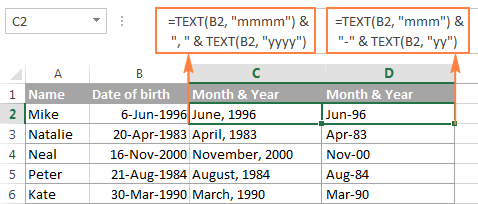### Example 3. Display a date as a year

If it does not really matter how the dates are stored in your workbook, you can get Excel to show only the years without changing the original dates. In other words, you can have full dates stored in cells, but only the years displayed.

In this case, no formula is needed. You just open the Format Cells dialog by pressing Ctrl + 1, select the Custom category on the Number tab, and enter one of the below codes in the Type box:

• yy - to display 2-digit years, as 00 - 99.
• yyyy - to display 4-digit years, as 1900 - 9999.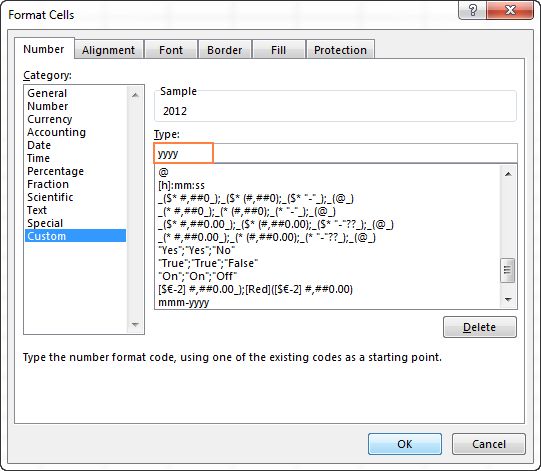Please remember that this method does not change the original date, it only changes the way the date is displayed in your worksheet. If you refer to such cells in your formulas, Microsoft Excel will perform date calculations rather than year calculations.

You can find more details about changing the date format in this tutorial: How to change date format in Excel.

## How to calculate age from date of birth in Excel

There are several ways to calculate age form date of birth in Excel - using DATEDIF, YEARFRAC or INT function in combination with TODAY(). The TODAY function supplies the date to calculate age at, ensuring that your formula will always return the correct age.

### Calculate age from date of birth in years

The traditional way to calculate a person's age in years is to subtract the birth date from the current date. This approach works fine in everyday life, but an analogous Excel age calculation formula is not perfectly true:

INT((TODAY()-DOB)/365)

Where DOB is the date of birth.

The first part of the formula (TODAY()-B2) calculates the difference is days, and you divide it by 365 to get the number of years. In most cases, the result of this equation is a decimal number, and you have the INT function round it down to the nearest integer.

Assuming the date of birth is in cell B2, the complete formula goes as follows:

`=INT((TODAY()-B2)/365)`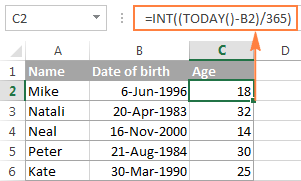As mentioned above, this age calculation formula is not always flawless, and here's why. Every 4th year is a leap year that contains 366 days, whereas the formula divides the number of days by 365. So, if someone was born on February 29 and today is February 28, this age formula will make a person one day older.

Dividing by 365.25 instead of 365 is not impeccable either, for example, when calculating the age of a child who hasn't yet lived through a leap year.

Given the above, you'd better save this way of calculating age for normal life, and use one of the following formulas to calculate age from date of birth in Excel.

DATEDIF(DOB, TODAY(), "y")
ROUNDDOWN(YEARFRAC(DOB, TODAY(), 1), 0)

The detailed explanation of the above formulas is provided in How to calculate age in Excel. And the following screenshot demonstrates a real-life age calculation formula in action:

`=DATEDIF(B2, TODAY(), "y")`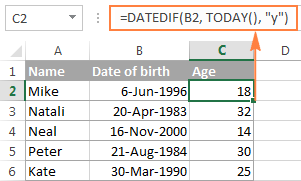### Calculating exact age from date of birth (in years, month and days)

To calculate an exact age in years, months and days, write three DATEDIF functions with the following units in the last argument:

• Y - to calculate the number of complete years.
• YM - to get the difference between the months, ignoring years.
• MD - to get the difference between the days, ignoring years and months.

And then, concatenate the 3 DATEDIF functions in a single formula, separate the numbers returned by each function with commas, and define what each number means.

Assuming the date of birth is in cell B2, the complete formula goes as follows:

`=DATEDIF(B2,TODAY(),"Y") & " Years, " & DATEDIF(B2,TODAY(),"YM") & " Months, " & DATEDIF(B2,TODAY(),"MD") & " Days"`

This age formula may come in very handy, say, for a doctor to display the exact age of patients, or for a personnel officer to know the exact age of all employees: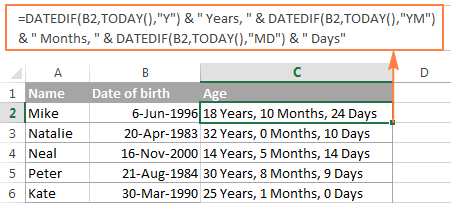For more formula examples such as calculating age at a particular date or in a certain year, please check out the following tutorial: How to calculate age in Excel.

## How to get the day number of the year (1-365)

This example demonstrates how you can get the number of a certain day in a year, between 1 and 365 (1-366 in leap years) with January 1 considered day 1.

For this, use the YEAR function together with DATE in this way:

`=A2-DATE(YEAR(A2), 1, 0)`

Where A2 is a cell containing the date.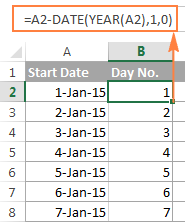And now, let's see what the formula actually does. The YEAR function retrieves the year of the date in cell A2, and passes it to the DATE(year, month, day) function, which returns the sequential number that represents a certain date.

So, in our formula, `year` is extracted from the original date (A2), `month` is 1 (January) and `day` is 0. In fact, a zero day forces Excel to return December 31 of the previous year, because we want January 1 to be treated as the 1st day. And then, you subtract the serial number returned by the DATE formula from the original date (which is also stored as a serial number in Excel) and the difference is the day of the year you are looking for. For example, January 5, 2015 is stored as 42009 and December 31, 2014 is 42004, so 42009 - 42004 = 5.

If the concept of day 0 does not seem right to you, you can use the following formula instead:

`=A2-DATE(YEAR(A2), 1, 1)+1`

## How to calculate the number of days remaining in the year

To compute the number of days remaining in the year, we are going to use the DATE and YEAR functions again. The formula is based on the same approach as Example 3 above, so you are unlikely to have any difficulties with understanding its logic:

`=DATE(YEAR(A2),12,31)-A2`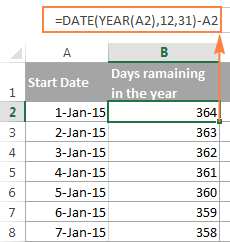If you want to know how many days remain till the end of the year based on the current date, you use the Excel TODAY() function, as follows:

`=DATE(2015, 12, 31)-TODAY()`

Where 2015 is the current year.

## Calculating leap years in Excel

As you know, nearly every 4th year has an extra day on February 29 and is called a leap year. In Microsoft Excel sheets, you can determine whether a certain date belongs to a leap year or a common year in a variety of ways. I'm going to demonstrate just a couple of formulas, which in my opinion are easiest to understand.

#### Formula 1. Check if February has 29 days

This is a very obvious test. Since February has 29 days in leap years, we calculate the number of days in month 2 of a given year and compare it with number 29. For example:

`=DAY(DATE(2015,3,1)-1)=29`

In this formula, the DATE(2015,3,1) function returns the 1st day of March in the year 2015, from which we subtract 1. The DAY function extracts the day number from this date, and we compare that number with 29. If the numbers match, the formula returns TRUE, FALSE otherwise.

If you already have a list of dates in your Excel worksheet and you want to know which ones are leap years, then incorporate the YEAR function in the formula to extract a year from a date:

`=DAY(DATE(YEAR(A2),3,1)-1)=29`

Where A2 is a cell containing the date.

The results returned by the formula are as follows: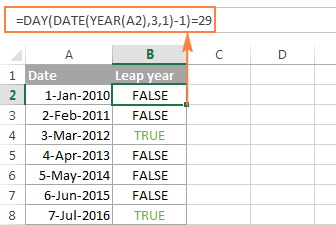Alternatively, you can use the EOMONTH function to return the last day in February, and compare that number with 29:

`=DAY(EOMONTH(DATE(YEAR(A2),2,1),0))=29`

To make the formula more user-friendly, employ the IF function and have it return, say, "Leap year" and "Common year" instead of TRUE and FALSE:

`=IF(DAY(DATE(YEAR(A2),3,1)-1)=29, "Leap year", "Common year")`

`=IF(DAY(EOMONTH(DATE(YEAR(A2),2,1),0))=29, "Leap year", "Common year")`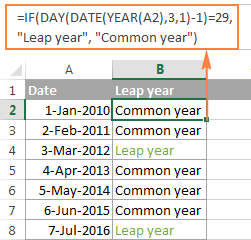#### Formula 2. Check if the year has 366 days

This is another obvious test that hardly requires any explanation. We use one DATE function to return 1-Jan of the next year, another DATE function to get 1-Jan of this year, subtract the latter from the former and check if the difference equals to 366:

`=DATE(2016,1,1) - DATE(2015,1,1)=366`

To calculate a year based on a date entered in some cell, you use the Excel YEAR function exactly in the same way as we did in the previous example:

`=DATE(YEAR(A2)+1,1,1) - DATE(YEAR(A2),1,1)=366`

Where A2 is a cell containing the date.

And naturally, you can enclose the above DATE / YEAR formula in the IF function for it to return something more meaningful than the Boolean values of TRUE and FALSE:

`=IF(DATE(YEAR(A2)+1,1,1) - DATE(YEAR(A2),1,1)=366, "Leap year", "Non-leap year")`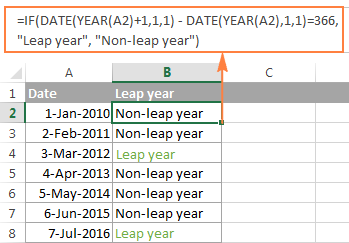As already mentioned, these are not the only possible ways to calculate leap years in Excel. If you are curious to know other solutions, you can check the method suggested by Microsoft. As usual, Microsoft guys are not looking for easy ways, are they?

Hopefully, this article has helped you figure out year calculations in Excel. I thank you for reading and look forward to seeing you next week.

## You may also be interested in

1. Eg. I need formula that the text function for 26th January 2023 to 25th February 2023 , result is February 2023.
What function should I use ?

• I also want to know?

2. Hii, I just want to check that if I am putting date as 01 Jan 2020 and I have to add the 3 years and I want the answer has 31 Dec 2023 please provide formula to calculate

3. Is it possible to format a year (just the number) as a date? I tried using the yyyy, but it calculates a specific date. For instance, the cell has the year 2003, if I format it using the yyyy, it will show the stored date 6/25/1905. I need it for a csv file to create a dashboard and have the key date. Thank you!

• Hi!
If the cell contains only the year 2003, then this is a number, not a date. You can convert a year number to a date using the DATE function.
For example,

DATE(2003,1,1) or DATE(A1,1,1)

4. Suppose you have a date in a cell (A1): 3/14/2020 and in another cell (A2) you need to return 12/31/2032 the end of year date 10 years from A1. I have the formula for to get the 10 years from now, but can't figure out how to get the 12/31 aspect of it without saying the month differential which I prefer to avoid because A1 date is not a constant.

• Edit: A2 should return 12/31/2030... not 12/31/2032

5. 7 years,11 months, 24 days Is given value in a1. I want to use if formula with if a1>20,12,24. How to use the tool. Kindly guide

6. Hi,

I'm working on a spreadsheet which contains data for 2020, however halfway through the spresdsheet excel has changed the year to 2021, Can this be fixed apart from manually going through the spreadshet and updating each date maunally?

• Hello!
You can add a year to 2020 dates in a separate column. Then copy these results into your original date column.

=IF(YEAR(A1)=2020,DATE(2021,MONTH(A1),DAY(A1)),A1)

7. I want to make a sheet for employees leave calculation. So in that if the employee joining date last year 19 december 2021 in one column i need to bring same day and month but current year how can i do it.

• Hi!
To get the desired date, use the DATE function. Determine the current year using the YEAR and TODAY() functions:

=DATE(YEAR(TODAY()),MONTH(A1),DAY(A1))

8. how to split this 06/03/1971 (50 years old ) to this 06/03/1971 only with fuction??

9. How can i sort in ascending order in this format 0 years, 0 months, 29 days?

• Hello!
Your data is text. For correct sorting, I recommend that you write down all numbers with two digits. For example

01 years, 05 months, 09 days

10. Hi,

I want to know about " when employee complete 58 year from DOB , How I can calculate ?

Is there any formula for that?

11. Hi,
I am having a problem with the TODAY() function. When I input =INT((Today()-D5)/365) all I get is a date ending in the year 1900, rather than the person's age. What is going wrong please?
Thanks.

12. Is there a formula for:

“7/01/2021 - 6/30/2022”

• Hi!
I am not sure I fully understand what you mean.

13. It becomes even worse: It doesn't look as if Excel can do dates before 1900? I cannot attach a screen shot here, can I?

• Hi Christine,

You are right - Excel does not recognize dates before 1900. In the internal Excel system dates are stored as serial numbers beginning with January 1, 1900, which is stored as 1.

14. Oh, and I cannot invent days to get around this, either, because the program abbreviates both, the 1900s and the 2000s to the last 2 digits, so there is no longer a difference between the two millenia (i.e. 1913 and 2013 both show up only as 13 - Why??). Not so with the 1800, it would still be 1813 - what IS going on??

• Hi,
To display the year in four digits, use the "yyyy" format. If you only want the year in the date, write the date as 01-01-1913.

15. This is bizarre... Is there no way just to enter simple years into an Excel table without converting from something, so that Excel recognizes it as a year??
I made a simple data table and a line chart with two data sets. But... They came out as separate, consecutive curves, not overlapping. And the years with data were not spread in a time scale in my graph, but regularly, even where I had 100-year gaps. I decided it is, because the cells were formatted as "general". OK, but whatever I tried messed up my year values. I don't have days to start with, only years. I reformatted the cells and re-entered the years, and they change. I. e. I type in 1818, and the cell makes 1904 out out of it (Why??). Same happens if I type the data in first, then reformat to yyyy. If I invent dates before reformatting, all ends up as the same year. This is so wrong. I wouldn't bother with the cell format and leave it at "general", but then Excel doesn't get the distances right between the time periods. Can Excel not do years by themselves???

• Hello!
If I understood the problem correctly, you want to work with dates before 1900. Unfortunately, Excel only works with dates after January 1, 1900.

16. Hi,
How to find the date of birth by retirement date and retirement age?
For Example: Retirement date is 01-Mar-2014 and Retirement age is 58 years.
Now how to find the date of birth.

17. Can some one help me to hide values in zero if I use this formula to calculate employment tenure in organization.
Example.
I get the result 0 years 0 months 20 days.
I want to show it as 20 days.

Regards.

• Hello!
The information you provided is not enough to understand your case and give you any advice, sorry.
What formula you used? Give an example of the source data.
It’ll help me understand it better and find a solution for you.

• I used the mentioned below formula to calculate employment tenure in years months and days.

=DATEDIF(B2,TODAY(),"Y") & " Years, " & DATEDIF(B2,TODAY(),"YM") & " Months, " & DATEDIF(B2,TODAY(),"MD") & " Days"

18. I'd like to calculate end-dates for financial purposes.

E.g., a security with a 1 year maturity period will mature on 31/12/2020, if the investment was made on 01/01/2020.

DATE formulae in Excel that I have seen so far seem to reflect the anniversary of the start date, i.e., 1 year from 01/01/2020 is given as 01/01/2021 (and not 31/12/2020) and 5 years from 01/01/2020 is given as 01/01/2025 (and not 31/12/2024, which is the correct date).

Is there any way to address this?

19. I have list of employees with their date of birth (in dd-mmm-yyyy format) n a worksheet.
I want to highlight the date of birth in rows which are unique. ie., just the date & month when they are unique ignoring the year. eg., there may be two employees, 1 with date of birth 2-Jun-1993 & another with date of birth 2-Jun-1975. So, i want these two to be highlighted ignoring the year.

• Hello!
I recommend using an extra column. Write down the dates of birth with the same year in it. You can use something like this:

=DATE(2000,MONTH(A1),DAY(A1))

In this column use conditional formatting - Highlight cell rules - Duplicate Values

20. Hello,
i want to determine and the remarks appear based on below in one cell only
if date is less than 6 months from now = SLOWLY MOVING
if date is less than 1 year from now = NON MOVING
if date is less than 3 months = RUNNING

21. I only have a data of year and number of day in a year. Example Year is 2020 and the number of day in the year is 32 then the result should be Feb 2, 2020. Is there a formula which I can obtain this result?

• Correction: the result should be Feb 1, 2020

22. Would appreciate if you give provide a formula for below scenario. Thank you in advance.
Start date: 5-27-2013
End Date: 10-3-2019
Counting number of years using a fraction of at least six (6)months being considered as one (1) whole year. So, 5-27-2013 to 12-31-2013, is considered one (1) year. Counting on a yearly basis, it means that from 5-27-2013 to 10-3-2019, is considered 7 years. Can you please provide me a formula for these?
Regards,
Felix

23. Doj - 01-07-2005
27-01-2013
03-01-2000
cut off date - 01-04.2019
How many year experience (made hiffen in all dates)
Manjunath

24. mere pass age ka column hai....date of year define karna hai kaise hoga..
example
srno name age dob( dd-mm-yyyy)
1 aaa 42
2 bbbb 55
3 ccc 65
4 ddd 32

25. how can I use if function to calculate the YEAR column from a given date column? Example :I HAVE A COLUMN
23/06/2018
13/07/2017
6/08/2001
24/10/2006
GENERATE ANOTHER COLUMN FOR YEAR USING IF FUNCTION ?
I NEED THE FORMULAR PLS?
THANKS

26. Hello
I need to calculate the number of days that is in each month that regards to the Start date and End date.
Fx. Des 18 (zero), Jan 19 (zero), feb 19 (13 days) , mar 19 (31 days) and so on

Start date ---- End date des.18 jan.19 feb.19 mar.19 apr.19 mai.19 jun.19
15.02.2019 18.10.2019

27. Hi, I hope you can help me with the correct formula to calculate separation pay. What is the formula to show that a fraction of at least six (6) months shall be considered as one (1) whole year.

Start Date: 02/08/1995
Separation Date: 09/05/2018
Years of Service: 23 years and 6 months

How can I get 24 years total years of service since 6 months should be considered an additional year? Thankful for your help in advance.

28. I was wondering how i can add year to date to an excel sheet gor payroll i have no current year to date on the spreadsheet right now?

• April:
I think you're asking how to create a running total. This is where the latest data is added to the existing total to display the most current total. This procedure is repeated each time data is added so that the sheet shows the newest data and the newest total.
If this is what you want to do, you create a running total by entering a range formula which is part absolute reference and part relative reference. The range formula looks like this: \$A\$1:A1. Absolute reference with the "\$", relative reference without it. Where A1 is the first cell in the range.
So, if the cell that will hold the payroll to be used in the Year-to-Date amount is in column "D" and the calculated running total is in column "E" the formula in column "E" will look like this:
=SUM(\$D\$6:D6) formula in E6 displays Year-to-Date of amounts in cells above E6
=SUM(\$D\$6:D7) formula in E6 displays Year-to-Date of amounts in cells above E7
=SUM(\$D\$6:D8) formula in E6 displays Year-to-Date of amounts in cells above E8
After you've copied it down to row 6, 7 & 8. This will calculate the running total to row 8. Just continue to copy this formula down the "E" column to display the most current Year-to-Date.

29. How can i add year to date for payroll?

30. Hi

I have tenure as 17.5 years, need to know the joining date from today what formula I should use.

Regards,
Ashish

31. Hi ,

If I have 13/05/2014 date and I want to convert to Financial year April to March how do I do?
Thanks.

32. Hello experts! I wish to calculate a student's current grade in school based on their graduation year. The trick is that students' grade levels change as of July 1st each year. Examples: A student's graduation year is 2021. Today is May 25, 2018. They are currently in 9th grade, but as of July 1st, 2018 will be in 10th grade. Perhaps it would be useful to assume that "graduation year X" really equals "June 30, year X"?

Here are the graduation years and corresponding grade levels through June 30th. As of July 1st, the grade level will need to rise by 1.

2029 - 1
2028 - 2
2027 - 3
2026 - 4
2025 - 5
2024 - 6
2023 - 7
2022 - 8
2021 - 9
2020 - 10
2019 - 11
2018 - 12

Further, could the formula cause the cell to be shaded yellow if the grade is 1-5, green if grade 6-8, blue if grade 9-12, and pink if grad?

It would also be useful to be able to do the opposite - calculate a grad year based on their current grade in school.

I know you must enjoy a good puzzle!

Many thanks.
Stuart R

33. Hi,

I want to know how to calculate retirement age remaining years in negative value means if employees already cross the retirement date so how they show in reaming year in negative value.

34. Hi All,

I have one query regarding my data I have a cell-like 05;11;18 and where 05, 11 and 18 are the date so how can I extract and count it as 3.

35. Hi All,

How can I add year fraction in excel. for e.g. I have 1st company experience as 2.6 (2 years 6 months) and next company experience is 16.10 (16 years 10 months) if I am adding both ideally my total experience is 19.4 (19 years 4 months) whereas if I am trying to calculate in excel, outcome is 18.76.

Please advise if I could use any alternate formula to sum up year fraction in excel.

Regards,
Vikram

• Hello,

=(2+16+TRUNC((6+10)/12)) &" years " & ((6+10)-(12*TRUNC((6+10)/12))) & " months"

36. Hi,

I want to calculate service period in three different cell at excel. Ex- Suppose someone's service year is 05 Years, 10 Months & 09 days. I want to show above three result in three different cell. Is it possible? if possible please reply me. I am real big trouble.

Thanks
Asfaq

37. What is the formula I should use when trying to find the total number of entries for a specific year? See Below:
First Name Rejection Date
Laura 12/17/2013
Veronica 12/19/2013
Jonathan 1/23/2014
Erin 2/1/2014
Lucinda 2/24/2015
Rosa 3/14/2015
Maria 7/30/2016
David 8/7/2017
Cheryl 8/7/2017
Paige 8/7/2017

I am trying to figure out how many people were "rejected" in 2013, 2014, 2015, etc. without having to create numerous columns, etc.

• Hi, Megan,

38. I want to get the length of service of my manpower. Is there a formula for this in excel? So that I could identify the number of years of my manpower. Thank you

39. Thanks, this helped me a lot.
Regards,
Js

40. Hi
how to change 10-mar-40 to 10-mar-2040 in excel

• Hi,

After you entered the date (make sure to enter the complete year - 2040, not just 40), select the cell and press Ctrl+1 to open the Format Cells window. There, go to Date tab and find the following data type: 14-Mar-2012. Select it and press OK.

Hope it helps!

41. Hi
Help!
How can I exit 35 months in Excel?

1/1/1988 TO 31/121988
1/1/1989 TO 31/12/1989
1/1/1990 TO 30/11/1990

42. Hi
Help!
aHow can I exit 35 months in Excel?

1/1/1988 TO 31/121988
1/1/1989 TO 31/12/1989
1/1/1990 TO 30/11/1990

43. Formula for calculating when a person will be 17 years and 6 months old?

44. using the formula =DATEDIF(A2,NOW(),"y") gets me the age for A2. how do I put that formula into 300 rows with out having to change the formula each it each time i.e:
=DATEDIF(A3,NOW(),"y")
=DATEDIF(A4,NOW(),"y")
=DATEDIF(A5,NOW(),"y")
etc.

Thanks

45. Hi
Help!
I need a formula that will calculate the number of days in the year over a period of time, not from January to December, that will take into account leap years. For example a date of contract starts 01/02/2016 and ends 31/01/2017 = 366 days. But, a contract that starts 01/04/2016 and ends 31/03/2017 is 365 days. How do I create a formula that will take this into consideration across several years and lines of data?
Thanks :)

46. I want to calculate Date of Birth of 25 years 4 months 12 days to date/month/year(ex.01/01/2017) format, kindly help, thanks.

47. trying to figure out the date. if age 18 or more at time of exam, then purge date is 7 years from date of exam.

If under 18 on date of exam, then purge date is 7 years from 18th birthday.

I Have one student
DOB 10/2/1985
Exam 03/07/04

Second Student
11/01/1960
06/12/78

and about 700 other students. how do I find the purge date?

• DOB Exam Date Age@exam Purge date
11/01/60 06/12/1978 17 ?